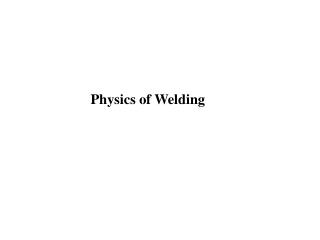DownloadDownload PresentationPhysics of Welding

# Physics of Welding

Télécharger la présentation## Physics of Welding

- - - - - - - - - - - - - - - - - - - - - - - - - - - E N D - - - - - - - - - - - - - - - - - - - - - - - - - - -
##### Presentation Transcript

1. Physics of Welding

2. Physics of Welding • Learning Activities • Read Handbook pp 32-62 • Look up Keywords • View Slides; • Read Notes, • Listen to lecture • Do on-line workbook • Do homework • Lesson Objectives • When you finish this lesson you will understand: • Heat input and heat transfer from arc to weld • Metal melting and regions of weld. • Arc physics and plasma properties of arc Keywords: Heat Input, Heat Transfer Efficiency, Heat Affected Zone, Enthalpy of Melting, Latent Heat, Melting efficiency, Plasma, Polarity, Thermionic Work Function, Ionization, Cathode Spot, Anode Spot, Arc I-V Characteristics

3. Physics of Welding • Heat Input Concepts • Energy Sources • Arc Characteristics • Wire Melting

4. Heat Input Heat Loss Heat input

5. Heat Input H = energy input, energy/unit length, joules /mm H = Power/Travel Speed, = P/v P = total input power, Watts v = travel speed of heat source, mm/sec Describes energy per unit length delivered, not rate of delivery Used in codes & specifications This energy does not all go entirely to the work

6. Heat Input for Arcs H = P/v = EI/v E = Arc Voltage (Volts) I = Arc Current (Amps) EI = Process power, converted to Heat v = Welding Travel Speed Not all the arc energy goes into the work Hnet = f1H = f1P/v = f1EI/v f1 = Heat Transfer Efficiency

7. f1 = Heat Transfer Efficiency short Arc Length long

8. Reinforcement Melted Base Metal Heat Affected Zone Aw = Cross Section of Weld = Am + Ar For Autogenous Weld (no filler metal) Aw = Am Heat Required to elevate solid to MP + Latent Heat of Fusion Heat Required to melt a Given Volume of Weld = Q =

9. Enthalpy of Melting Heat Required to elevate solid to MP + Latent Heat of Fusion Heat Required to melt a Given Volume of Weld = Q = Not all the net heat transferred goes into melting

10. Melting Efficiency f2 = Melting efficiency, the fraction of the process heat energy per unit length delivered to the metal which is required to melt the metal f2 = QAw/Hnet f2 = QAwv/f1EI From previous slide: Hnet = f1H = f1P/v = f1EI/v • Melting Efficiency Depends On: • Higher Thermal Conductivity - Lower Efficiency • High Energy Density Heat Source - Higher Efficiency

11. Questions? • Turn to the person sitting next to you and discuss (1 min.): • We can select a range of processes for arc welding from a tiny GTAW run at 15 volts and 100 amps and 30 ipm to twin arc submerged arc welds run at 25 volts and total curretn of over 1000 amps run at 8 ipm. What is the heat input in each of these welds? What do you think might happen to the cooling rate in the part being welded when the weld is stopped in each of these weld?

12. Other Energy Sources H = Heat generated, joules E = Voltage, volts v = Travel Speed, mm/sec I = Current, amps R = Resistance, ohms t = Time, sec PD = Power Density P1 = Input power Arc H = EI/v Resistance: H = I2Rt Electroslag: H = EIt Laser: EB: A = Area of focused beam

13. OXYFUEL GAS WELDING THERMIT WELDING

14. Homework Do Homework Assignment 4 “Physics of Welding” From the Assignment page of the WE300 Website. Often engineering calculations require conversion of units. In the “Slide Show Mode”, clicking on this icon will open a free program to help you with conversions. You might want to bookmark this program for later use as well. On your first use, please click on help and register this free program.

15. Arc Characteristics

16. Polarity and Current Flow Welding Electrode or "Electrode" Anode Cathode I I DCEP DCEN Cathode Anode Work Electrode or "Work" Reverse RPEP Straight SPEN

17. Plasma State Gas is hot enough so that high energy collisions produce free electrons Plasma may only be a few % electrons

18. Conduction of Current in the Arc Cathode Electrons Emitted Thermal Free Ionization Electron Ion Plasma T>10,000K Recombination Neutral Anode Gas Atom Electrons Absorbed

19. Argon Arc

20. Thermionic Work Function Energy Required for electron to escape a solid surface

21. Work Function of pure Tungsten = 4.4 eV Work Function of Thoriated W = 4.1 eV

22. Ionization Free "Neutral" I Electron Atom Ionization Collision Free Ion Free Electron with Energy > Ionization Potential Ionization Potentials: He 24.6 eV Ar 15.8 N 15.6 Fe 7.9 Na 5.1

23. } } } Will total voltage change if we change the amount of current (say from 200 amps to 300 amps)?

24. Arc V-I Characteristic Unstable We see that current and arc length have an effect, what happens if we change from Ar to some other ionizing gas?

25. Ionization Potential He 24.6 ev Ar 15.8 N 15.6 Fe 7.9 Na 5.1 P 4.3

26. Questions? • Turn to the person sitting next to you and discuss (1 min.): • The arc characteristics that we looked at were for a Gas Tungsten Arc where the electrode is not melted so the metal ions in the arc do not come from molten electrode. What happens in GMAW where the wire (electrode) melts? Would you expect anything different to happen?